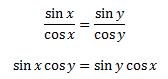# Trigonometric Equations

The equations that involve the trigonometric functions of a variable are called trigonometric equations. In the upcoming discussion, we will try to find the solutions of such equations. These equations have one or more trigonometric ratios of unknown angles. For example, cos x -sin2 x = 0, is a trigonometric equation which does not satisfy all the values of x. Hence for such equations, we have to find the values of x or find the solution.

We know that sin x and cos x repeat themselves after an interval of 2π, and tan x repeats itself after an interval of π. The solutions such trigonometry equations which lie in the interval of [0, 2π] are called principal solutions. A trigonometric equation will also have a general solution expressing all the values which would satisfy the given equation, and it is expressed in a generalized form in terms of ‘n’. The general representation of these equations comprising trigonometric ratios is;

E1(sin x, cos x, tan x) = E2(sin x, cos x, tan x)
Where E1 and E2 are rational functions.

Since sine, cosine and tangent are the major trigonometric functions, hence the solutions will be derived for the equations comprising these three ratios. However, the solutions for the other three ratios such as secant, cosecant and cotangent can be obtained with the help those solutions.

## Solutions for Trigonometric Equations

Let us begin with a basic equation, sin x = 0. The principal solution for this case will be x = 0, π, 2π as these values satisfy the given equation lying in the interval [0, 2π]. But, we know that if sin x = 0, then x = 0, π, 2π, π, -2π, -6π, etc. are solutions of the given equation. Hence, the general solution for sin x = 0 will be, x = nπ, where n∈I.

Similarly, general solution for cos x = 0 will be x = (2n+1)π/2, n∈I, as cos x has a value equal to 0 at π/2, 3π/2, 5π/2, -7π/2, -11π/2 etc. Below here is the table defining the general solutions of the given trigonometric functions involved equations.

 Equations Solutions sin x = 0 x = nπ cos x = 0 x = (nπ + π/2) tan x = 0 x = nπ sin x = 1 x = (2nπ + π/2) = (4n+1)π/2 cos x = 1 x = 2nπ sin x = sin θ x = nπ + (-1)nθ, where θ ∈ [-π/2, π/2] cos x = cos θ x = 2nπ ± θ, where θ ∈ (0, π] tan x = tan θ x = nπ + θ, where θ ∈ (-π/2 , π/2] sin2 x = sin2 θ x = nπ ± θ cos2 x = cos2 θ x = nπ ± θ tan2 x = tan2 θ x = nπ ± θ

### Proof of Solutions of Trigonometric Equations

Now let us prove these solutions here with the help of theorems.

Theorem 1: For any real numbers x and y, sin x = sin y implies x = nπ + (–1)n y, where n ∈ Z

Proof: Consider the equation, sin x = sin y. Let us try to find the general solution for this trigonometric equation.

sin x = sin y

⇒ sin x – sin y = 0

⇒2cos (x + y)/2 sin (x – y)/2 = 0

⇒cos (x + y)/2 = 0 or sin (x – y)/2 = 0

Upon taking the common solution from both the conditions, we get:

x = nπ + (-1)ny, where n ∈ Z

Theorem 2: For any real numbers x and y, cos x = cos y, implies x = 2nπ ± y, where n ∈ Z.

Proof: Similarly, the general solution of cos x = cos y will be:

cos x – cos y = 0

2sin (x + y)/2 sin (y – x)/2 = 0

sin (x + y)/2 = 0 or sin (x – y)/2 = 0

(x + y)/2 = nπ or (x – y)/2 = nπ

On taking the common solution from both the conditions, we get:

x = 2nπ± y, where n ∈ Z

Theorem 3: Prove that if x and y are not odd mulitple of π/2, then tan x = tan y implies x = nπ + y, where n ∈ Z.

Proof: Similarly, to find the solution of equations involving tan x or other functions, we can use the conversion of trigonometric equations.

In other words, if tan x = tan y then;sin x cos y – sin y cos x = 0

sin (x – y) = 0     [By trigonometric identity]

Hence, x – y =nπ or x = nπ + y, where n ∈ Z.

### Trigonometric Equations Examples

Let us go through an example to have a better insight into the solutions of trigonometric equations.

Q.1 : sin 2x – sin 4x + sin 6x = 0

Solution:Given: sin 2x – sin 4x + sin 6x = 0

⇒sin 2x + sin 6x – sin 4x = 0

⇒2sin 4x.cos 2x – sin 4x = 0

⇒sin 4x (2cos 2x – 1) = 0

⇒sin 4x = 0 or cos 2x = ½

⇒4x = nπ or 2x = 2nπ ± π/3

Therefore, the general solution for the given trigonometric equation is:

⇒x = nπ/4 or nπ ± π/6

Q.2: Find the principal solution of the equation sin x = 1/2.

Solution: Since we know, sin π/6 = 1/2

and sin 5π/6 = sin (π – π/6) = sin π/6 = 1/2

Therefore, the principal solutions are x =π/6 and x = 5π/6.